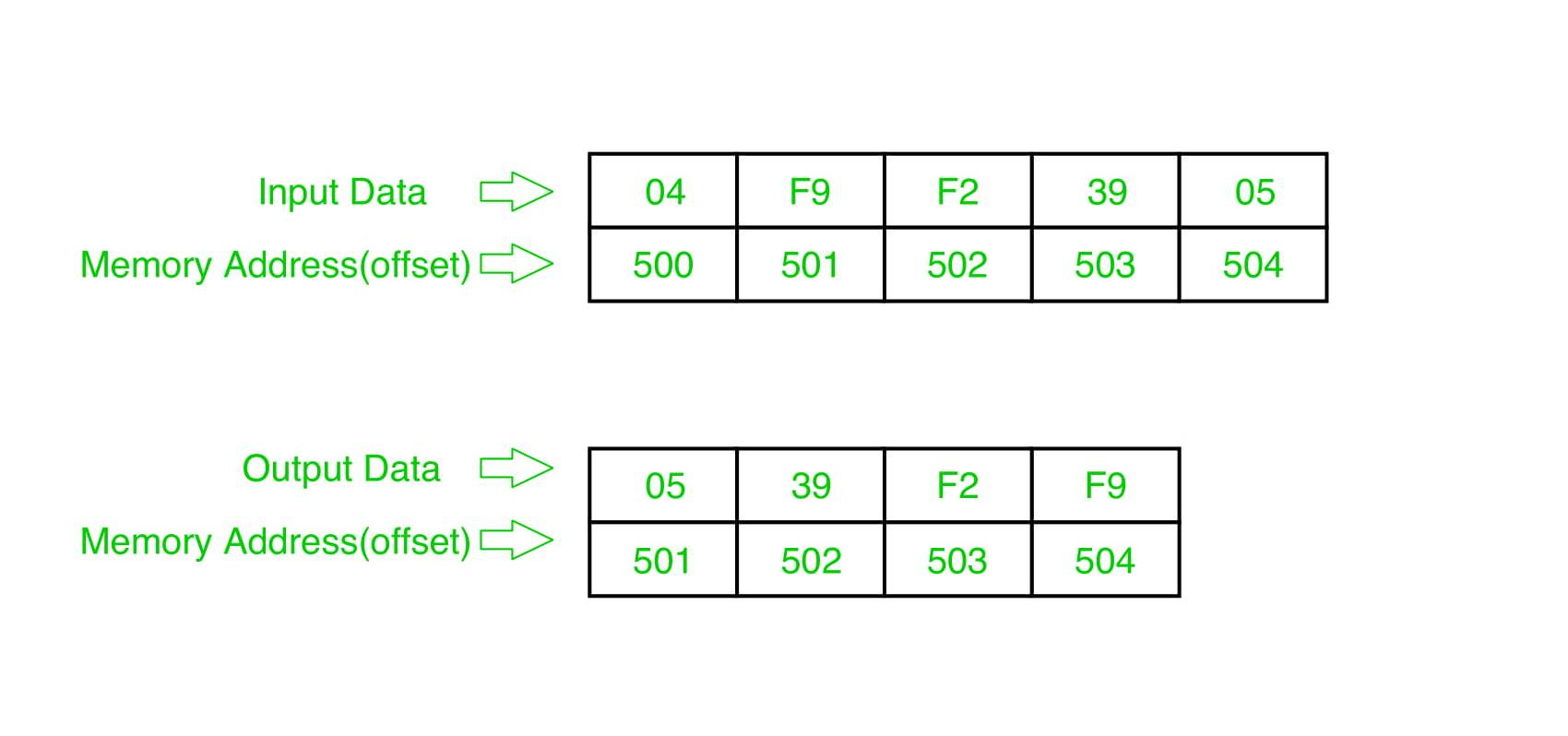Related Articles
8086 program to sort an integer array in ascending order
• Difficulty Level : Medium
• Last Updated : 22 May, 2018

Problem – Write a program in 8086 microprocessor to sort numbers in ascending order in an array of n numbers, where size “n” is stored at memory address 2000 : 500 and the numbers are stored from memory address 2000 : 501.

Example –Example explanation:

```Pass-1:
F9 F2 39 05
F2 F9 39 05
F2 39 F9 05
F2 39 05 F9 (1 number got fix)

Pass-2:
F2 39 05 F9
39 F2 05 F9
39 05 F2 F9 (2 number got fix)

Pass-3:
39 05 F2 F9
05 39 F2 F9 (sorted)```

Algorithm –

1. Load data from offset 500 to register CL (for count).
2. Travel from starting memory location to last and compare two numbers if first number is greater than second number then swap them.
3. First pass fix the position for last number.
4. Decrease the count by 1.
5. Again travel from starting memory location to (last-1, by help of count) and compare two numbers if first number is greater than second number then swap them.
6. Second pass fix the position for last two numbers.
7. Repeate.

Program –

MEMORY ADDRESSMNEMONICSCOMMENT
400MOV SI, 500SI<-500
403MOV CL, [SI]CL<-[SI]
405DEC CLCL<-CL-1
407MOV SI, 500SI<-500
40AMOV CH, [SI]CH<-[SI]
40CDEC CHCH<-CH-1
40EINC SISI<-SI+1
40FMOV AL, [SI]AL<-[SI]
411INC SISI<-SI+1
412CMP AL, [SI]AL-[SI]
414JC 41CJUMP TO 41C IF CY=1
416XCHG AL, [SI]SWAP AL AND [SI]
418DEC SISI<-SI-1
419XCHG AL, [SI]SWAP AL AND [SI]
41BINC SISI<-SI+1
41CDEC CHCH<-CH-1
41EJNZ 40FJUMP TO 40F IF ZF=0
420DEC CLCL<-CL-1
422JNZ 407JUMP TO 407 IF ZF=0
424HLTEND

Explanation –

1. MOV SI, 500: set the value of SI to 500.
2. MOV CL, [SI]: load data from offset SI to register CL.
3. DEC CL: decrease value of register CL BY 1.
4. MOV SI, 500: set the value of SI to 500.
5. MOV CH, [SI]: load data from offset SI to register CH.
6. DEC CH: decrease value of register CH BY 1.
7. INC SI: increase value of SI BY 1.
8. MOV AL, [SI]: load value from offset SI to register AL.
9. INC SI: increase value of SI BY 1.
10. CMP AL, [SI]: compares value of register AL and [SI] (AL-[SI]).
11. JC 41C: jump to address 41C if carry generated.
12. XCHG AL, [SI]: exchange the contents of register AL and SI.
13. DEC SI: decrease value of SI by 1.
14. XCHG AL, [SI]: exchange the contents of register AL and SI.
15. INC SI: increase value of SI by 1.
16. DEC CH: decrease value of register CH by 1.
17. JNZ 40F: jump to address 40F if zero flat reset.
18. DEC CL: decrease value of register CL by 1.
19. JNZ 407: jump to address 407 if zero flat reset.
20. HLT: stop.

Attention reader! Don’t stop learning now. Get hold of all the important CS Theory concepts for SDE interviews with the CS Theory Course at a student-friendly price and become industry ready.

My Personal Notes arrow_drop_up